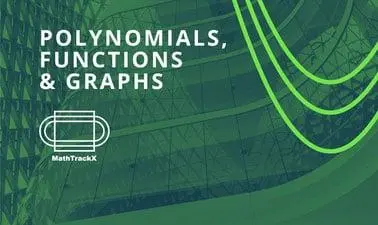There is 0 item now in your comparison listView a comparison list

# MathTrackX: Polynomials, Functions and Graphs

Price
Free
10,107 people completed this program

## Overview

This course is part one of the MathTrackX XSeries Program which has been designed to provide you with a solid foundation in mathematical fundamentals and how they can be applied in the real world.

This course will lay down the foundations of basic mathematical vocabulary and play a role in communicating key concepts throughout the MathTrackX Program. A central concept underpinning this course is the mathematical concept of function. Functions occur throughout mathematics and an understanding of them is essential.

Guided by experts from the School of Mathematics and the Maths Learning Centre at the University of Adelaide, this course will introduce functions, the algebra of numbers & polynomials and sets of numbers and intervals of the real number line.

• Algebra and arithmetic
• Basic vocabulary for sets of real numbers
• Basic concepts of functions
• Understanding and graphing polynomials
• Mathematical problem solving

## Authored by